# Electromagnetic Induction

## Homework Statement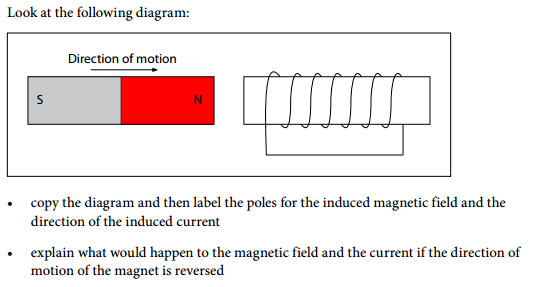## Homework Equations

no equations required

3. The Attempt at a Solution
so here are my answers, can some please verify if these are correct. Thanks in advance :)
a)
i have no idea if im labelling the poles correctly, or if the induced current is in the right direction , any help would be appreciated!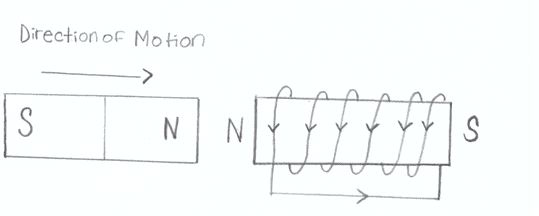b)

If the direction of motion of the magnet is reversed, in this case, instead of the magnet moving towards the coil, the magnet will pull away from the coil, the field of the magnet will decrease in strength. Reversing the direction of motion of the magnet also affects the current induced within the coil. Reversing the direction of the magnet reverses the direction of the current in the coil. I drew a diagram to demonstrate what would happen to the magnetic field of the magnet and the current induced when the direction of motion of the magnet is reversed: is this right?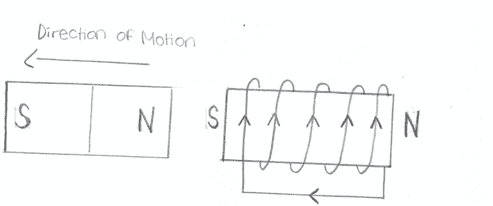according to the law of lenz, a changing magnetc field will cause a current to be induced in a conductor, this conductor will have an induced magnetic field that will oppose the change that caused it. So for a) , as the magnet moves towards the conductor, its magnetic field is increasing in strength, this will induce a current and a magnetic field that will oppose the permanent magnet. That is why i made the left side of the conductor N-pole, so it can repel the magnet coming towards it, is this correct? Should the current in the conductor be going the opposite way?

and for part b), since the magnetic field of the conductor must repel the change that caused it, the left-side of the conductor should be s-pole, so it can repel the magnet that is moving away from the conductor. so does the current direction of the conductor have to be reversed??

Is it the poles or the current direction that im getting wrong in both part a) and part b)??
can you be more specific?

#### Attachments

Your intuition is right, but use the right hand rule and see if it matches the current directions you've chosen.

SammyS
Staff Emeritus
Homework Helper
Gold Member
according to the law of lenz, a changing magnetc field will cause a current to be induced in a conductor, this conductor will have an induced magnetic field that will oppose the change that caused it. So for a) , as the magnet moves towards the conductor, its magnetic field is increasing in strength, this will induce a current and a magnetic field that will oppose the permanent magnet. That is why i made the left side of the conductor N-pole, so it can repel the magnet coming towards it, is this correct? Should the current in the conductor be going the opposite way?

and for part b), since the magnetic field of the conductor must repel the change that caused it, the left-side of the conductor should be s-pole, so it can repel the magnet that is moving away from the conductor. so does the current direction of the conductor have to be reversed??

Is it the poles or the current direction that im getting wrong in both part a) and part b)??
can you be more specific?
I believe that is what axmis was saying. Your choice of direction for current produces a magnetic polarity opposite of what you show. (This assumes you are referring to conventional current rather than the direction of electron current.)

ok so i used the right hand rule and this is what i got:
so for a) the current is supposed to be the opposite way around: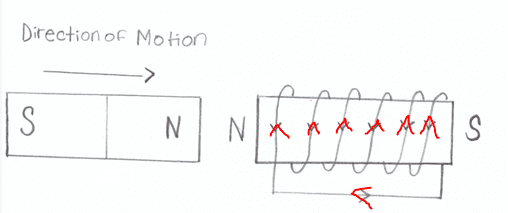and for b) the direction of the current is opposite to what i drew initially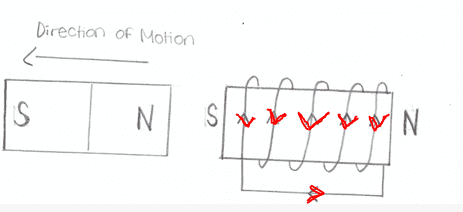is this right?

Correct. So, your poles are correct. You just needed to realize that only one direction of current would actually give you that pole configuration, and you utilize the right hand rule to figure out what direction that is.

ok just to clarify, the final answer that i posted is correct!
and thanks for the help